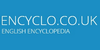### calculation1. problem solving that involves numbers or quantities
2. planning something carefully and intentionally

### CalculationA calculation is a deliberate process that transforms one or more inputs into one or more results, with variable change. The term is used in a variety of senses, from the very definite arithmetical calculation of using an algorithm, to the vague heuristics of calculating a strategy in a competition, or calculating the chance of a successful relati...
Found on http://en.wikipedia.org/wiki/Calculation

### Calculation• (n.) The act or process, or the result, of calculating; computation; reckoning, estimate. • (n.) An expectation based on circumstances.
Found on http://thinkexist.com/dictionary/meaning/calculation/

### calculation(from the article `chemical bonding`) There are two strands of approach to the computation of molecular structure. In the semiempirical approach, the calculation draws on a number of ... The development of new methods of numerical calculation was a response to the increased practical demands of numerical computation, particularly in ......
Found on http://www.britannica.com/eb/a-z/c/6

### calculationnoun planning something carefully and intentionally; `it was the deliberation of his act that was insulting`
Found on https://www.encyclo.co.uk/local/20974

### calculationcomputation noun the procedure of calculating; determining something by mathematical or logical methods
Found on https://www.encyclo.co.uk/local/20974

### Calculation[card game] Calculation (also known as Broken Intervals) is a solitaire card game played with a standard pack of 52 cards. It offers more scope for skill than many similar games; a skilled player can win Calculation more than half of the time when `normal play` can allow winning 1 in 5 times. At the start of play, an ace, two, three, and fo...
Found on http://en.wikipedia.org/wiki/Calculation_(card_game)

### Calculation[game] Falone (22.1ppg, 6.6rpg) ...
Found on http://en.wikipedia.org/wiki/Calculation_(game)

### CalculationCal`cu·la'tion (-lā'shŭn) noun [ Middle English calculation , from Latin calculatio ; confer Old French calcucation .] 1. The act or process, or the result, of calculating; computation; reckoning, estimate. 'The calculation of eclipses.' Nichol.
Found on http://www.encyclo.co.uk/webster/C/8

### calculationdetermining something by mathematical or logical methods
Found on https://www.vocabulary.com/lists/310886

### calculation[n] - problem solving that involves numbers or quantities 2. [n] - planning something carefully and intentionally 3. [n] - the procedure of calculating
Found on http://www.webdictionary.co.uk/definition.php?query=calculation
No exact match found